## 《高等数学》22春平时作业1-00001

《高等数学》22春平时作业1-00001

------------------------
1.函数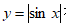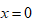处的连续性和可导性是（ ）
A.连续、可导
B.不连续、不可导
C.不连续、可导
D.连续、不可导

2.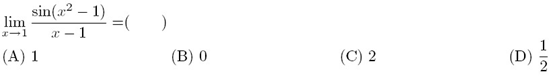A.(A)
B.(B)
C.(C)
D.(D)

3.题面见图片A.A
B.B
C.C
D.D

4.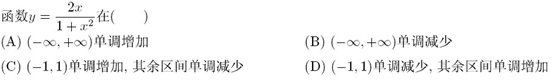A.(A)
B.(B)
C.(C)
D.(D)

5.题面见图片A.A
B.B
C.C
D.D

6.设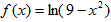，则函数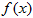的连续区间是（ ）
A.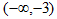B.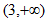C.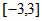D.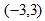7.设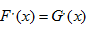，则（ ）
A.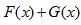为常数
B.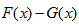为常数
C.D.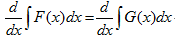8.设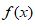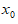可导且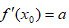，则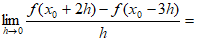（ ）
A.0
B.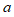C.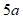D.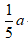9.题面见图片A.A
B.B
C.C
D.D

10.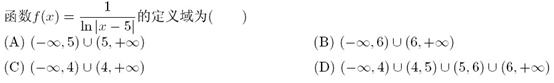A.(A)
B.(B)
C.(C)
D.(D)

11.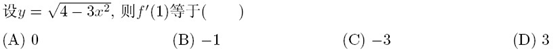A.(A)
B.(B)
C.(C)
D.(D)

12.题面见图片A.A
B.B
C.C
D.D

13.题面见图片A.A
B.B
C.C
D.D

14.设函数，则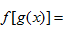（　）
A.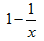B.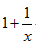C.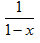D.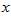15.设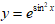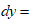（ ）
A.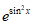B.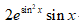C.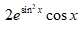D.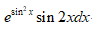16.题面见图片A.A
B.B
C.C
D.D

17.题面见图片A.A
B.B
C.C
D.D

18.下列极限存在的是（ ）．
A.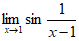B.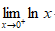C.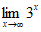D.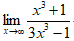19.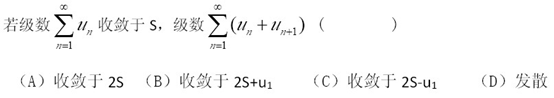A.(A)
B.(B)
C.(C)
D.(D)

20.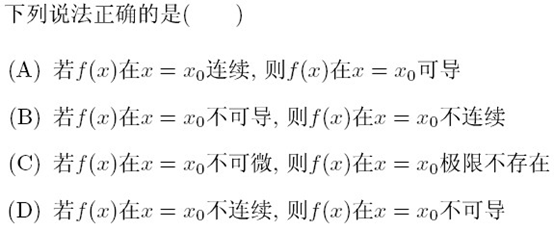A.(A)
B.(B)
C.(C)
D.(D)